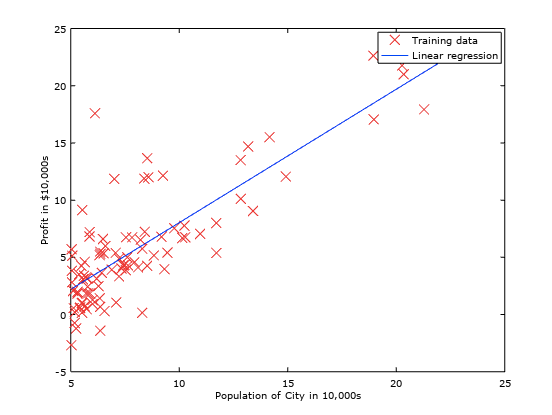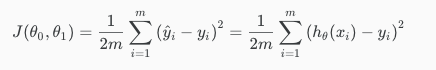Categories

# Machine Learning Notes — Week 1

#### Definition of Machine Learning:

The field of study that gives computers the ability to learn without being explicitly programmed.

In other words, a Computer program is said to learn from experience E with respect to some class of tasks T and performance measure P, if its performance at tasks in T, as measured by P, improves with experience E.

#### Supervised Learning:

In supervised learning, we are given a data set and already know what our correct output should look like, having the idea that there is a relationship between the input and the output.

Regression: trying to predict results within a continuous output, meaning that we are trying to map input variables to some continuous function. Eg: predicting housing prices given no. of bedrooms, area etc.Linear regression for Predicting profits based on population of a city

Classification: trying to predict results in a discrete output or trying to map input variables into discrete categories. Eg: Cat vs Dog classifier.

#### Unsupervised Learning:

In unsupervised learning, we approach ideas with little or no idea about what our results should look like. For example, Take a collection of 1,000,000 different genes, and find a way to automatically group these genes into groups that are somehow similar or related by different variables, such as lifespan, location, roles, and so on.

#### Model and Cost Function:

Given a training set, our goal is to learn a ‘hypothesis’ function h : X →Y, so that h(x) is a ‘good’ predictor for the corresponding value of y. It can be pictorially represented as follows:

We can measure the accuracy of our hypothesis function by using a cost function. This takes an average difference of all the results of the hypothesis with inputs from x’s and the actual output y’s. This is called the squared error function or mean squared error.Cost function J, where m is the number of training examples